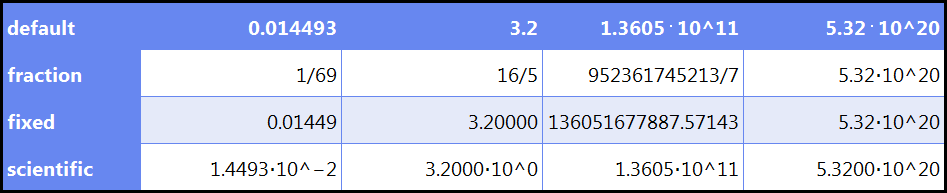﻿ SetNumberFormat – Algosim documentation
Algosim documentation: SetNumberFormat

# SetNumberFormat

Sets the number format of a numeric entity.

## Syntax

• `SetNumberFormat(x, fmt)`

• `x` is a number, a vector, or a matrix

• `fmt` is a string

## Description

`SetNumberFormat(x, fmt)` returns `x` with the number format set to `fmt`.

`fmt` is one of the following values:

• `"inherited"`

The global setting will be used.

• `"default"`

Standard format. Very large and very small numbers are displayed in scientific (exponential) notation, and only such numbers. Insignificant zeros are not included.

• `"fraction"`

Same as `"default"` except that rational numbers are written as the quotient of two integers instead of using decimal notation.

• `"fixed"`

Numbers are written without scientific notation if at all possible and if so with a fixed number of digits after the decimal separator. Trailing zeros in the fractional part are used, if necessary, to achieve this.

• `"scientific"`

All numbers are written in scientific notation with exactly one digit before the decimal separator and a fixed total number of digits. Trailing zeros are used if necessary.

## Examples

`L ≔ '(1/69, 16/5, 952361745213/7, 5.32E20); F ≔ '("default", "fraction", "fixed", "scientific");`
`ToTable(F @ (f ↦ '(f) ~ L @ (x ↦ SetNumberFormat(x\5, f))))`